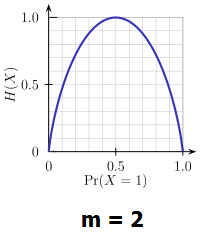# Current Slide

Small screen detected. You are viewing the mobile version of SlideWiki. If you wish to edit slides you will need to use a larger device.

### Brief Review of Entropy

• Entropy (Information Theory)
• A measure of uncertainity associated with a random variable
• Calculation: for a discrete random variable Y taking m distinct values {y1,...,ym},
$H(Y)=-\sum_{i=1}^{m}p_{i}log(p_{i}), p_{i}=P(Y=y_{i})$
• Interpretation:
• Higher entropy=>higher uncertainty
• Lower entropy=>lower uncertainty
• Conditional entropy
$H(Y|X)=\sum_{x}p(x)H(Y|X=x)$Speaker notes:

## Content Tools

### Sources

There are currently no sources for this slide.